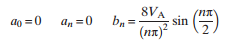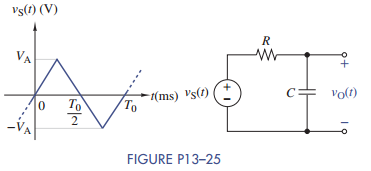### Create an Account

Home / Questions / The periodic triangular wave in Figure P13–25 is applied to the RC circuit shown in the f...

# The periodic triangular wave in Figure P13–25 is applied to the RC circuit shown in the figure The Fourier coefficients of the input are If VA 10 V and T0 2π ms

The periodic triangular wave in Figure P13–25 is applied to the RC circuit shown in the figure. The Fourier coefficients of the input areIf VA = 10 V and T0 = 2π ms, find the first four nonzero terms in the Fourier series of vO (t) for R =5kΩ and C = 0.01 μF.May 24 2020 View more View LessSubscribe To Get Solution